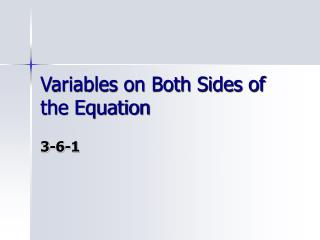DownloadDownload PresentationVariables on Both Sides of the Equation

# Variables on Both Sides of the Equation

Télécharger la présentation## Variables on Both Sides of the Equation

- - - - - - - - - - - - - - - - - - - - - - - - - - - E N D - - - - - - - - - - - - - - - - - - - - - - - - - - -
##### Presentation Transcript

1. Moving terms • When a one step equation is solved, essentially the constants are sorted to one side of the equation and the variable to the other. • Think of this as “moving” the constant 8 to the other side of the equation.

2. Moving terms • “Move” terms involving variables the same way. • Either the 3x or the 5x could be moved. • Choose the term containing the variable with the smaller coefficient. • This doesn’t matter now, but will make things easier in other equations. • Now there is only a two step equation to solve.

3. Steps • To solve equations with variables on both sides of the equal sign, • 1- Distribute. • 2- Combine like terms. • 3- Move all the variables to the same side. • 4- Solve the two step equation. • Subtract or add • Divide. • Every step isn’t needed in every equation.

4. Steps • To solve equations with variables on both sides of the equal sign, • 1- Distribute. • 2- Combine like terms. • 3- Move all the variables to the same side. • 4- Solve the two step equation. • Subtract or add • Divide. • Every step isn’t needed in every equation.